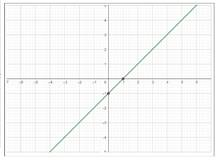# To graph : using a graphing calculator.### Precalculus: Mathematics for Calcu...

6th Edition
Stewart + 5 others
Publisher: Cengage Learning
ISBN: 9780840068071### Precalculus: Mathematics for Calcu...

6th Edition
Stewart + 5 others
Publisher: Cengage Learning
ISBN: 9780840068071

#### Solutions

Chapter 2.3, Problem 9E

a.

To determine

## To graph: using a graphing calculator.

Expert Solution

### Explanation of Solution

Given information: consider the provided function

f(x)=x1

Graph:

Step 1: Open Graphing Calculator from the start menu.

Step 2: Click on the input box and enter the function provided x1

Step 3: Press Enter.

Hence, the graph so obtained is,b.

To determine

### To calculate: the domain and the range from the graph

Expert Solution

the domain from the graph is [,] and the range from the graph is [,] .

### Explanation of Solution

Given information: consider the graph obtained in part a.,Calculation:

The domain of the function h is all the ix-values of the points on the graph, and the range is all the corresponding y -values.

Since, the graph obtained in part a. is straight line and has infinite number of x and y values the domain is [,] and the range is [,]

Hence, the domain from the graph is [,] and the range from the graph is [,] .

### Have a homework question?

Subscribe to bartleby learn! Ask subject matter experts 30 homework questions each month. Plus, you’ll have access to millions of step-by-step textbook answers!